# Mathematical Modelling of Electric Field Generated by Vertical Grounding Electrode in Horizontally Stratified Soil Using the FDTD Method

Mathematical Modelling of Electric Field Generated by Vertical Grounding Electrode in Horizontally Stratified Soil Using the FDTD Method
Electrical Engineering and Plasmas Laboratory, Ibn Khaldoun University of Tiaret, Tiaret 14000, Algeria

APELEC Laboratory, Djilali Liabès University of Sidi Bel-Abbès, Sidi Bel-Abbes 22000, Algeria

Corresponding Author Email:
abdelber.bendaoud@univ-sba.dz
Page:
251-257
|
DOI:
https://doi.org/10.18280/mmep.070211
27 February 2020
|
Accepted:
13 May 2020
|
Published:
30 June 2020
| Citation

OPEN ACCESS

Abstract:

The aim of this work is to evaluate the electric fields generated by vertical grounding electrode in horizontally stratified soil during the passage of the lightning current through the grounding rod. The computation of the electric fields is performed using the Finite-Difference Time-Domain method in three dimensions (FDTD-3D). The observation points are located under and above ground. The effect of multi-layers soil on the transient behavior of the vertical grounding electrode and the associated electric field is illustrated and discussed. The obtained results were firstly compared and validated with other ones published in the literature for the case of homogeneous ground. The used method calculates easily the transients of grounding rod; it gives certain flexibility when taking into account the stratification of the soil and gives a better visualization of the electromagnetic field radiation. The found results can be easily used for the electromagnetic coupling problems.

Keywords:

FDTD, transient grounding, electric field, electromagnetic compatibility, stratified soil

1. Introduction

During a lightning strike, a large impulse current can flow in the grounding systems causing a large transient voltage along the buried electrode. The induced voltage and current surge can generate in the electric and electronic apparatus, connected or not to the grounding systems, electromagnetic Interferences (EMI). These Interferences can cause malfunctions, operation errors, destructions of electronic equipment and so on; moreover transient step and touch voltages are dangerous for people. For all these reasons, the study of the grounding systems excited by a high impulse current due to a lightning strike is useful to check their performances and also it is helpful for practical aims. A lot of analytical and numerical models are proposed in the literature in order to analyse the grounding systems under lightning strikes. They are mainly based on:

(1) The Transmission Lines Approach,

(2) The circuit approach,

(3) The hybrid approach,

(4) The electromagnetic fields approach.

Sunde  was perhaps the first one who introduced the transmission line concept with frequency dependent per-unit length parameters for modelling the transient behavior of single horizontal grounding wire on the surface of the soil due to direct lightning strikes using telegrapher’s equations.

In the 1980’s, Verma et al. have applied the concept of lossy transmission lines to a horizontal ground wire by solving analytically the telegrapher’s equations in frequency domain [2, 3]. The passage from frequency domain to time domain requires the inverse Fourier transform.

Later, Lorentzou et al.  started from the same equations to calculate current and voltage distribution on the wire directly in the time domain. Transmission line approach can predict surge propagation delay, which becomes important when the grounding system has large size. Further, the computational time required for transmission line approach is extremely less compared to the electromagnetic field approach.

The circuit approach was introduced by Meliopoulos and Moharam  in 1983 for analyzing the transient of grounding systems. The ground electrode was modelled by an equivalent circuit with lumped elements RLC, where different coupling modes (capacitive, inductive and conductance) between the ground conductors can be considered. Circuit approach can easily incorporate the non-linear soil ionization phenomena. Furthermore, circuit approaches can include all the mutual coupling between the grounding wires. The main drawback of this approach is that it cannot predict the surge propagation delay.

The hybrid approach was initiated by Dawalibi [6, 7] in 1986, and amended in 2000 by Andolfato et al.  to study grounding systems transient. The term “hybrid” means that this approach is a combination of the electromagnetic approach and the circuit approach. The merit of hybrid approach is that the frequency influence on series internal impedances, inductive components and capacitive-conductive components are included, which makes the above said approach more accurate than the conventional circuit approach, especially when the injection source frequency is high.

The last approach called electromagnetic fields approach is the most rigorous method for modeling the transient behavior of grounding systems. It solves the Maxwell equations with minimal approximations. This approach can be implemented either by the method of Moments (MoM), the Finite Element. Method (FEM) and the Finite-Difference Time-Domain method (FDTD). The MoM method was used for the first time, in the 1990’s, in the calculations of the transients in grounding systems by Grcev and Dawalibi [9, 10]. This method starts from electric field Maxwell’s integral equation. The FEM method as for it was introduced by Nekhoul et al. , in 1995, to simulate the behavior of the grounding systems. The model starts from electric or magnetic energy equations which involves partial differential Maxwell’s equations with respect to the vector potential (A) and the scalar potential (V) in different domains/volumes of the system. In 2001, Tanabe  performed experimental tests applying current pulses in large grounding electrodes. Auxiliary current and voltage electrodes have been employed to inject the current pulse and to collect voltage, respectively. With this experience, Tenabe validated his simulations based on FDTD method.

In 2009, Fortin et al.  have developed a calculation of the electric field radiated by a grounding grid in a stratified soil, using a full wave solution for the Hertz vector potential caused by an electric dipole located in the soil. The author's method can do DC calculations up to high frequencies with complex soil geometry. That is to say arbitrary soil parameters (layers number, resistivity and permittivity).

The objective of this work is to analyze the transient behavior of vertical grounding electrode in horizontally stratified soil during the passage of the lightning current through the grounding rod and then to evaluate the associated electric field. The computation of the electric fields is performed using the Finite-Difference Time-Domain method in three dimensions (FDTD-3D). The observation points are located above and underground. To the best of our knowledge, so far, there are no works in the literature dealing with the effect of soil stratification on the transient phenomena of the grounding systems.

This paper is organized as follows: In section II, the full-wave

FDTD technique is briefly described. In Section III, we present a description of thin wire representation. Section IV is devoted to the transient voltage calculation. In Section V, numerical simulations along with the relevant discussion are presented. Finally, in Section VI, general conclusions are drawn.

2. Finite-Difference Time-Domain Method

In the 1980’s, the development of computers gave engineers the possibility to solve the different equations governing physical phenomena using numerical methods. Among these methods, the FDTD is widely used for its advantages namely: resolution in three dimensions, describes easily different structures, possibility of introducing several materials, etc.

In 1966, Yee  introduced the first steps of this method which transform partial derivatives Maxwell’s Eqns. (1) and (2) to finite difference equations.

$\nabla \times H=\sigma E+\varepsilon \frac{\partial E}{\partial t}$     (1)

$\nabla \times E=-\mu \frac{\partial H}{\partial t}$     (2)

The Yee’s scheme consists the discretization of the computation domain into the small cubes of dimension ∆x, ∆y and ∆z, and writing Maxwell curl with FDTD. The expression of the fields E and H becomes:

$E_{x}^{n+1}\left(i+\frac{1}{2}, j, k\right)=k_{1} \cdot E_{x}^{n}\left(i+\frac{1}{2}, j, k\right)$

$+k_{2} \cdot\left[\begin{array}{c}\left(\frac{H_{z}^{n+\frac{1}{2}}\left(i+\frac{1}{2}, j+\frac{1}{2}, k\right)-H_{z}^{n+\frac{1}{2}}\left(i+\frac{1}{2}, j-\frac{1}{2}, k\right)}{\Delta y}\right) \\ +\left(\frac{H_{y}^{n+\frac{1}{2}}\left(i_{1}+\frac{1}{2}, j, k-\frac{1}{2}\right)-H_{y}^{n+\frac{1}{2}}\left(i+\frac{1}{2}, j, k+\frac{1}{2}\right)}{\Delta z}\right)\end{array}\right]$      (1a)

$H_{x}^{n+\frac{1}{2}}\left(i, j+\frac{1}{2}, k+\frac{1}{2}\right)$

$=k_{1} \cdot H_{x}^{n-1 / 2}\left(i, j+\frac{1}{2}, k+1 / 2\right)$

$+\frac{\Delta t}{\mu}\left[\begin{array}{c}\left(\frac{E_{y}^{n}\left(i, j+\frac{1}{2}, k+1\right)-E_{y}^{n}\left(i, j+\frac{1}{2}, k\right)}{\Delta z}\right) \\ +\left(\frac{E_{z}^{n}\left(i, j, k+\frac{1}{2}\right)-E_{z}^{n}\left(i, j+1, k+\frac{1}{2}\right)}{\Delta y}\right)\end{array}\right]$      (2a)

Coefficients k1 and k2 are given by:

$k_{1}=\frac{1-\frac{\sigma . \Delta t}{2 \varepsilon}}{1+\frac{\sigma \cdot \Delta t}{2 \varepsilon}}, k_{2}=\frac{\frac{\Delta t}{\varepsilon}}{1+\frac{\sigma \cdot \Delta t}{2 \varepsilon}}$ .

Other electromagnetic field components are deduced with the same manner.

From Eqns. (1a) and (2a) each component of the electric or magnetic field on the cube is surrounded by four components of the magnetic and electrical field, respectively, (Figure 1).

E is the electric field, H is the magnetic field; µ, σ, and ε are, respectively, the magnetic permeability, the electric conductivity, and the dielectric permittivity of the considered medium (air or ground).

## 1.png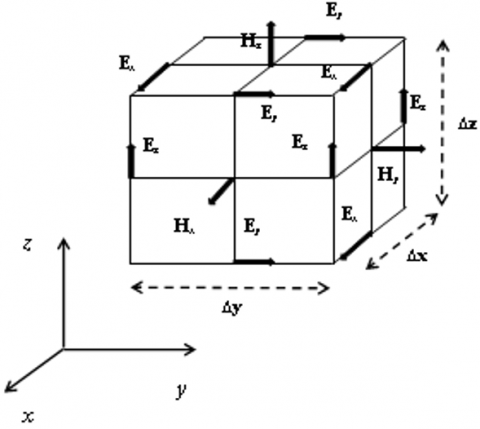Figure 1. Spatial Yee discretization grid

The FDTD algorithm requires specific considerations. The grid size should be a fraction of wavelength. In addition, to avoid numerical instabilities, the time increment should be determined satisfying the electric current’ stability criterion, namely,

$\Delta t \leq \frac{1}{C \cdot \sqrt{\frac{1}{(\Delta x)^{2}}+\frac{1}{(\Delta y)^{2}}+\frac{1}{(\Delta z)^{2}}}}$     (3)

where, c is the light celerity, ∆x, ∆y and ∆z special steps for axes x, y and z in Cartesian coordinate system.

The computational volume discretised must be truncated with the absorbing boundaries to minimize the parasitic reflections which can distort the results. In general the most used limits in the literature are: Mur, Liao’s, Perfect Matched Layer PML, Uniaxial Perfect Matched Layer UPML (Taflove's study ). In our case we have introduced the second-order Liao’s absorbing boundaries conditions because it functions well in the case of lossy materials, which corresponds to the ground being conductive .

3. Thin Wire Representation for FDTD Method

There are two techniques to represent a small radius relative to the smaller Yee cells: a) correction of tangential magnetic components near the wire with an analytical calculation of the magnetic field and then introducing it into the Maxwell equations . b) Correction of the electrical and magnetic parameters near the wire (Figure 2) to have an equivalent radius of 0.23.∆s. This technique is the most appropriate for a finite conductivity media containing small radius wires. For this, we adopted the second technique in this article. The corrected values of the electrical and magnetic parameters used for the calculation of the field components near the conductor are given by :

$\sigma^{*}=\sigma \frac{\ln (1 / 0.23)}{\ln (\Delta s / r)}$     (4)

$\varepsilon^{*}=\varepsilon \frac{\ln (1 / 0.23)}{\ln (\Delta s / r)}$     (5)

$\mu^{*}=\mu \frac{\ln (\Delta s / r)}{\ln (1 / 0.23)}$        (6)

The equivalent radius becomes 0.23.∆s after correction of parameters. Where r: radius of electrode; ∆s: spatial step.

The tangential component of the electric field along the conductor is equal to zero.

## 2.png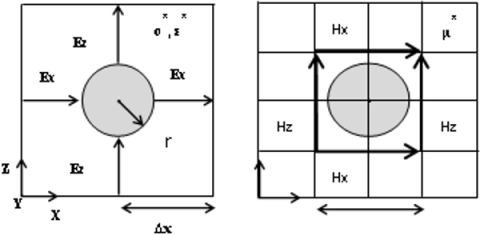Figure 2. Radial electric field and magnetic field of the thin wire

4. Transient Voltage

To have the transient voltage, there are two methods used in the literature. The first is to put a bar between a point near the injection point Va and the distant earth where the potential is equal to zero Vb (Figure 3a) . This way of doing is to bring the zero potential near the injection point in order to make the measurement. But this technique can influence the results because of induction. For this, we have resorted to another method which is based on the principle of the difference of the potential. To calculate the voltage between two points we can apply the Eq. (7), by using the integration of the electric field at the surface of the ground along the path perpendicular to the supply circuit from feed points to the absorbing limit, where the potential is equal to zero (Figure 3b) .

## 3.png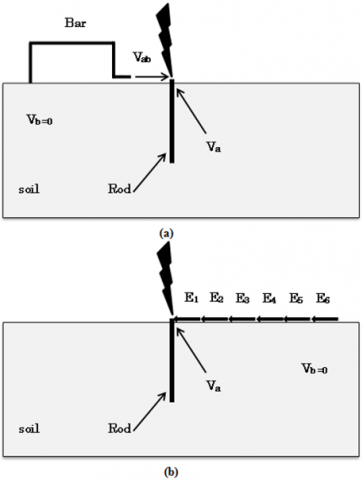Figure 3. Two technics to obtaining the transient voltage

$V=-\int_{a}^{b} E d l$     (7)

We can write with FDTD:

$V=-\sum_{N j}^{N k} E . \Delta s$     (8)

where: V is the voltage.

5. Results and Discussion

5.1 Behaviour of vertical grounding rod to a double peak bipolar current

Figure 4 shows the case that will be analysed with the FDTD method. A vertical earthing rod of 1 m length and 25 mm radius buried in a homogeneous soil of resistivity 42Ω.m and relative permittivity εr=10 fed by a current bipolar source, positive peak 30 kA and negative 10 kA (Figure 5). This configuration represents the experience made by Geri et al. . The working volume is 20m x 20m x20m divided on uniform cubes of 0.1m x0.1m x 0.1m surrounded by six layers of second order ABC Liao’s to minimize the reflections. The equivalent radius of the vertical grounding rod is 23mm (0.23Dx=0.23x0.1)  approximately the same electrode radius in the Geri experiment.

## 4.png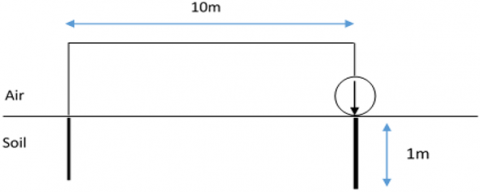Figure 4. Geri et al schema experiment

## 5.png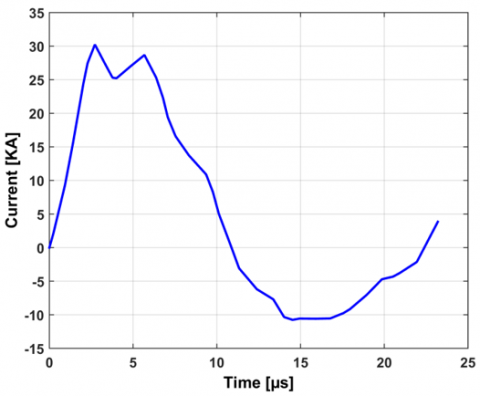Figure 5. Current injected in the top of grounding rod 

Figure 6 shows the transient voltage computed by the FDTD Method of a vertical grounding rod in a homogeneous soil. This voltage is obtained by integrating the tangential electric field at the surface of the ground from the feed point to the ABC limit. The obtained results are congruent with those obtained by Grcevet al. which validate our simulation code [21, 22].

## 6.pngFigure 6. Computed voltage at the top of grounding rod

Figure 7 presents the electric field radiated by the vertical grounding rod at three different points (p1, p2 and p3) above and underground. It can be seen that the electric field has the same shape at the observation points which is similar to the injected current wave.

## 7.png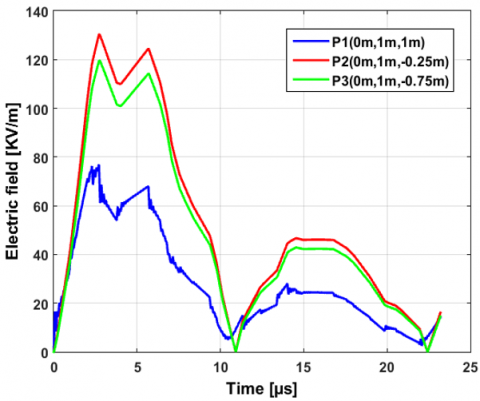Figure 7. Electric field radiated by grounding rod at three different observation points (p1, p2 and p3) in presence of homogeneous soil (ρ=42Ωm, εr=10)

5.2 Transient electric field radiated by a vertical grounding rod buried in a stratified soil with two layers

After validation of the obtained results, a study was carried out to see the influence of the stratified soil with two layers on the transient behaviour of the grounding rod and on the electric field radiated in each layer of the soil and in the air. Figure 9 presents the geometry of the problem. The vertical rod is powered by a lightning current which was used in the work of Grcev  (Figure 8).

## 8.pngFigure 8. Current injected in the top of grounding rod case of stratified soil 

## 9.png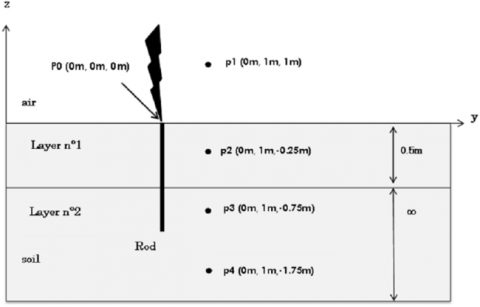Figure 9. Grounding rod buried in stratified soil (two layers)

The adopted values for the electrical parameters of the soil layers are given in Table 1. The depth of the upper layer was set to 0.5m (see Figure 9). Three cases were considered in the simulations: Case 1 corresponding to a homogeneous ground, while Cases 2 and 3 represent two conﬁgurations of two-layers soil. The horizontal soil stratiﬁcation was accounted simply by considering different values for the soil electrical parameters when passing from one grid point to another one belonging to a different layer.

Table 1. Electric parameters of the two-layer ground

 ρ1 (Ω.m) ρ 2 (Ω.m) εr Case 1 Homogeneous soil 42 42 10 Case 2 Stratiﬁed soil (ρ1<ρ2) 42 200 10 Case 3 Stratiﬁed soil (ρ1>ρ2) 200 42 10

In Figure 10, the voltage calculated at the point of impact of the rod for the homogeneous and stratified soil with 2 layers is displayed. It is easy to see the influence of the resistivity when a layer is added; when the apparent resistivity of the ground changes, the flow of the current to the earth changes, too. The high value of the resistivity increases the voltage of the electrode because of the weak dissipation of the current and vice versa.

## 10.png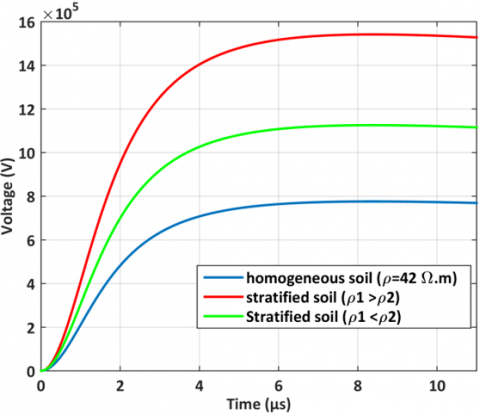Figure 10. Voltage at feed point of grounding rod

## 11.png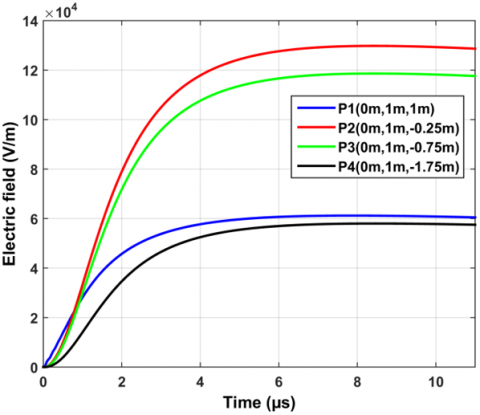Figure 11. Electric field radiated by grounding rod in homogeneous soil

## 12.png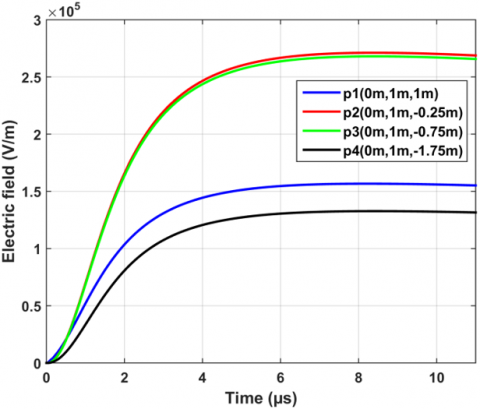Figure 12. Electric field radiated by grounding rod in stratified soil (ρ1=42Ω.m, ρ2=200Ω.m)

The radiated electric field was observed at four observation points p1, p2, p3 and p4, located respectively, at 1m above the ground, 0.25m, 0.75m and 1.75m below the ground (Figure 9).

From Figures 11-13, it can be seen that when the upper layer is more conductive than the lower one (case 2), the amplitude of electric field at all observation points p1, p2, p3 and p4 can increase respectively of 162, 107, 126 and 120 percent compared to those of homogeneous soil .For case 3 (ρ1>ρ2) the increase is about 27, 36, 26, 32 percent.

## 13.png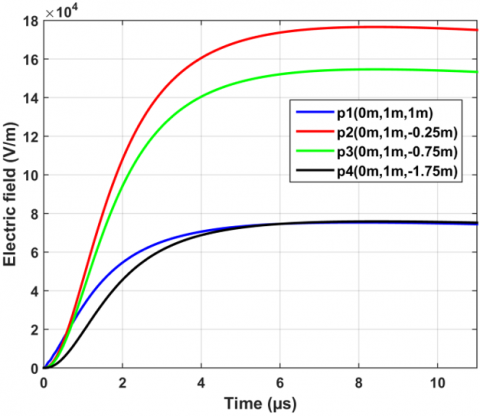Figure 13. Electric field radiated by grounding rod in stratified soil (ρ1=200 Ω.m, ρ2=42 Ω.m)

5.3 Transient current dissipated by a vertical grounding rod buried in a stratified soil with 2 layers

This part of the study is devoted to the dissipation of the current in the soil, for a homogeneous soil and stratified with two layers. Figure 14 shows the temporal currents which traverse the earth rod every 0.1 m from the point of impact (the highest graph) to the extreme (bottom graph). In this case the current distribution along the electrode is uniform due to the homogeneity of the soil.

## 14.png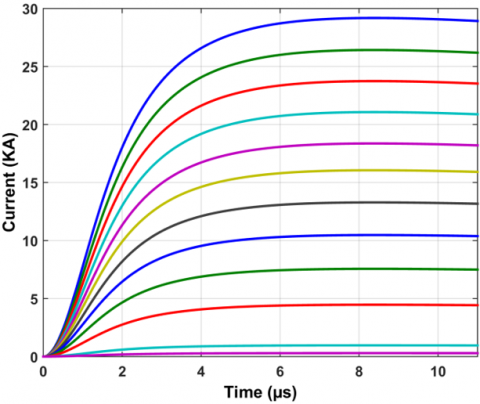Figure 14. Currents distribution along the grounding rod in homogeneous soil with a resistivity ρ=42Ω.m

Table 2. Grounding rod current comparison (homogeneous soil and 2 layers soil)

 0*l 0.2*l 0.4*l 0.6*l 0.8*l I(KA) (ρ=42Ω.m) 28.82 23.45 18.14 13.12 7.47 I1(KA) (ρ1<ρ2) 28.82 18.53 08.95 05.12 02.94 I2(KA) (ρ1>ρ2) 28.82 27.5 25.79 19.73 17.17 (I-I1)/I (%) 0,00 20,98 50,66 60,98 60,64 (I-I2)/I (%) 0,00 -17,27 -42,17 -50,38 -129,85

where, l is the length of the electrode, I the rod current in homogeneous soil case, I1 the rod current in stratified soil (case 2), I2 the rod current in stratified soil (case 3).

In order to have a clear idea about the current distribution along the earth electrode for a homogeneous and stratified soil, the results are given in Table 2 with a comparison of the different cases.

When the upper layer is more conductive than the lower one (case 2), the current decreases rapidly along the electrode with an average of 9 KA in the 1st layer and 3KA in the 2nd one.

## 15.png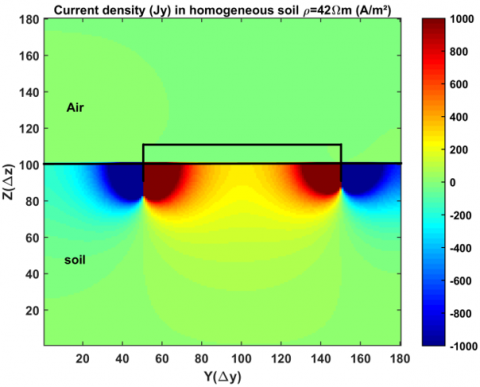Figure 15. Currents density in homogeneous soil ρ=42 Ω.m at t=11µs

## 16.png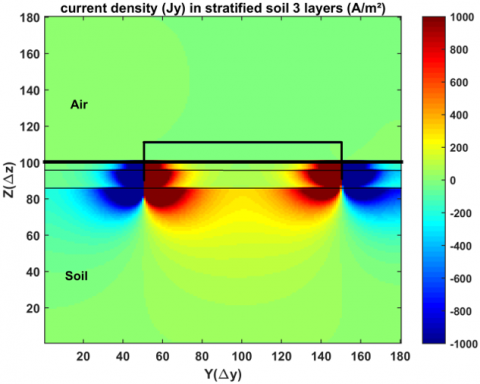Figure 16. Current density in a stratified soil in a stratified soil (ρ1=500 Ω.m, ρ2=200 Ω.m, ρ3=42 Ω.m) at t=11μs

## 17.png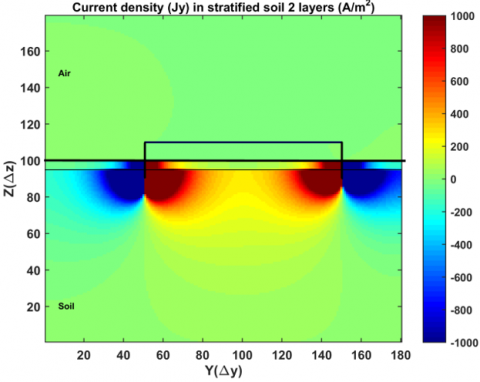Figure 17. Current density in a stratified soil in a stratified soil (ρ1=42 Ω.m, ρ2=200 Ω.m) at t=11μs

For the third case, when the lower layer is more conductive than the upper level, the current decreases slowly in the resistive layer along the rod with an average of 1.3 KA which justifies ohm's law.

The Figures e.g., Figures 15, 16 and 17, it is a cartography which reflects the results of the cases treated previously to give a better visibility of the flow of current through the different layers of the earth.

6. Conclusions

In this paper, we presented and discussed the effect of a horizontally stratiﬁed ground on the electric ﬁelds radiated by vertical grounding electrode during the passage of the lightning current through the grounding rod. The FDTD-3D technique was adopted for solving Maxwell’s time dependent equations directly in the time domain. The observation points are located under and above ground. The obtained results were firstly compared and validated with other ones published in the literature for the case of homogeneous ground.

A summary of conclusions obtained from the analysis are as follows:

The introduction of layers varies the apparent value of the soil.

A high value of the soil resistivity increases the value of the electric field in the soil and air, which implies an increase of the rod voltage and vice versa.

The current along the electrode is not homogeneous due to the different soil layers resistivity.

The earth electrode dissipates the current better in the least resistant layer.

The value of the current density is important near the electrode.

The results give a good view of the grounding transients, which facilitates understanding the phenomena.

The method used and the results obtained can be used in the case of electromagnetic coupling for the study of electromagnetic compatibility problems.

Nomenclature
 E Electric field, V.m-1 H Magnetic field A. m-1 V Electric potential, V t Time, s I Electric Current intensity, A l Length of the electrode c Celerity, m. s-1 r Radius, m Greek symbols ε Medium Permettivity, F. m-1 µ Medium Permeabilitty, H. m-1 ρ Medium resistivity, Ω.m σ Medium conductivity, S. m-1 ∆s Spatial step, m Subscripts a First point in space b Final point in space od Observation point Nj Inferior number of cell Nk Superior number of cell * Corrected values 1,2,3,4 Position
References

 Sunde, E.D. (1949). Earth Conduction Effects in Transmission Systems. Dover Publications Inc.

 Verma, R., Mukhedkar, D. (1980). Impulse impedance of buried ground wire. IEEE Transactions on Power Apparatus and Systems, PAS-99(5): 2003-2007. https://doi.org/10.1109/TPAS.1980.319827

 Mazzetti, C., Veca, G.M. (1983). Impulse behavior of ground electrodes. IEEE Transactions on Power Apparatus and Systems, PAS-102(9): 3148-3156. https://doi.org/10.1109/TPAS.1983.318122

 Lorentzou, M.I., Hatziargyriou, N.D., Papadias, B.C. (2003). Time domain analysis of grounding electrodes impulse response. IEEE Transactions on Power Delivery, 18(2): 517-524. https://doi.org/10.1109/TPWRD.2003.809686

 Meliopoulos, A.P., Moharam, M.G. (1983). Transient analysis of grounding systems. IEEE Transactions on Power Apparatus and Systems, PER-3(2): 389-399. https://doi.org/10.1109/MPER.1983.5519631

 Dawalibi, F. (1986). Electromagnetic fields generated by overhead and buried short conductors part 1 - single conductor. IEEE Transactions on Power Delivery, 1(4): 112-119. https://doi.org/10.1109/TPWRD.1986.4308036

 Dawalibi, F. (1986). Electromagnetic fields generated by overhead and buried short conductors part 2-ground networks. IEEE Transactions on Power Delivery, 1(4): 112-119. https://doi.org/10.1109/TPWRD.1986.4308037

 Andolfato, R., Bernardi, L., Fellin, L. (2000). Aerial and grounding system analysis by the shifting complex images method. IEEE Transactions on Power Delivery, 15(3): 1001-1009. https://doi.org/10.1109/61.871366

 Grcev, L., Dawalibi, F. (1990). An electromagnetic model for transients in grounding systems. IEEE Transactions on power Delivery, 5(4): 1773-1781. https://doi.org/10.1109/61.103673

 Grcev, L.D. (1996). Computer analysis of transient voltages in large grounding systems. IEEE Transactions on Power Delivery, 11(2): 815-823. https://doi.org/10.1109/61.489339

 Nekhoul, B., Guerin, C., Labie, P., Meunier, G., Feuillet, R., Brunotte, X. (1995). A finite element method for calculating the electromagnetic fields generated by substation grounding systems. IEEE Transactions on Magnetics, 31(3): 2150-2153. https://doi.org/10.1109/20.376472

 Tanabe, K. (2001). Novel method for analyzing the transient behavior of grounding systems based on the finite-difference time-domain method. 2001 IEEE Power Engineering Society Winter Meeting. Conference Proceedings (Cat. No. 01CH37194), 3: 1128-1132. https://doi.org/10.1109/PESW.2001.917230

 Fortin, S., Yang, Y., Ma, J., Dawalibi, F. (2009). Electromagnetic fields of energized conductors in multilayer medium with recursive methodology. 2009 Asia-Pacific Power and Energy Engineering Conference, Wuhan, China, pp. 1-4. https://doi.org/10.1109/APPEEC.2009.4918329

 Yee, K. (1966). Numerical solution of initial boundary value problems involving Maxwell's equations in isotropic media. IEEE Transactions on Antennas and Propagation, 14(3): 302-307. https://doi.org/10.1109/TAP.1966.1138693

 Taflove, A., Hagness, S.C. (2005). Computational electrodynamics: The Finite-Difference Time-Domain Method, Artech House. ISBN-10: 1580538320.

 Tanabe, K. (2001). Novel method for analyzing dynamic behavior of grounding systems based on the finite-difference time-domain method. IEEE Power Engineering Review, 21(9): 55-57. https://doi.org/10.1109/MPER.2001.4311614

 Umashankar, K.O.R. A.D.A.R., Taflove, A., Beker, B. (1987). Calculation and experimental validation of induced currents on coupled wires in an arbitrary shaped cavity. IEEE Transactions on Antennas and Propagation, 35(11): 1248-1257. https://doi.org/10.1109/TAP.1987.1144000

 Baba, Y., Nagaoka, N., Ametani, A. (2005). Modeling of thin wires in a lossy medium for FDTD simulations. IEEE Transactions on Electromagnetic Compatibility, 47(1): 54-60. https://doi.org/10.1109/TEMC.2004.842115

 Tuma, E.T., Dos Santos, R.O., de Oliveira, R.M., da Silva Souza, C.L. (2006). Transient analysis of parameters governing grounding systems by the FDTD method. IEEE Latin America Transactions, 4(1): 55-61. https://doi.org/10.1109/TLA.2006.1642450

 Geri, A., Veca, G.M., Garbagnati, E., Sartorio, G. (1992). Non-linear behaviour of ground electrodes under lightning surge currents: computer modelling and comparison with experimental results. IEEE Transactions on Magnetics, 28(2): 1442-1445. https://doi.org/10.1109/20.123966

 Grcev, L. (2009). Time-and frequency-dependent lightning surge characteristics of grounding electrodes. IEEE Transactions on Power Delivery, 24(4): 2186-2196. https://doi.org/10.1109/TPWRD.2009.2027511

 Otani, K., Shiraki, Y., Baba, Y., Nagaoka, N., Ametani, A., Itamoto, N. (2012, September). FDTD simulation of grounding electrodes considering soil ionization. In 2012 International Conference on Lightning Protection (ICLP), Vienna, pp. 1-6. https://doi.org/10.1109/ICLP.2012.6344295

 Grcev, L. (2009). Time-and frequency-dependent lightning surge characteristics of grounding electrodes. IEEE Transactions on Power Delivery, 24(4): 2186-2196. https://doi.org/10.1109/TPWRD.2009.202751